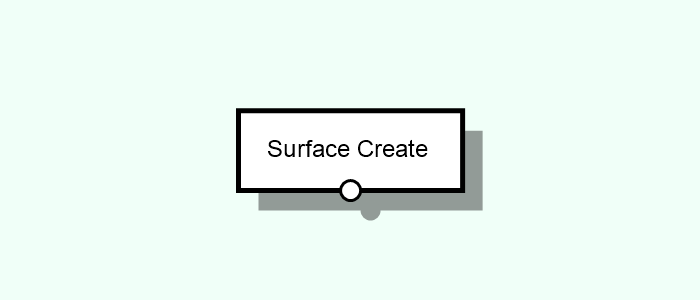# Surface Create

Creates or loads Surfaces with particular characteristics.Tags: Surface, Empty, From Mesh, Perlin, Ramp, Tileable

## Parameters#

Type [Select]
Type of surface pattern/method to create.
Empty [Compound]
Creates a empty, flat surface.
Width [Int]
Width (size in the X-Axis of the terrain.
Length [Int]
Length in the Y-Axis of the terrain.
Cell Size [Float]
Square size of each terrain cell. Should be a multiple of both Width and Length.
Interpolation [Choice]
The type of height data interpolation. Because surfaces are often rendered as triangle or quad meshes, there is more than one possible setup. Different target platforms may use different setups, which could affect, for instance, the positioning of the objects on the surface.
Top Left means that the quads are split from the top left corner to the bottom right corner.
Top Right means that the quads are split from the top right corner to the bottom left corner.
Bilinear assumes that the quad is not split and the height values are bilinearly interpolated.
From Mesh [Compound] [Adds ports 1]
Creates a surface from a mesh by sampling it uniformly in the XY plane.
Cell Size [Float]
Square size of each terrain cell. Should be a multiple of both Width and Length.
Interpolation [Choice]
The type of interpolation.
Perlin [Compound]
Creates a random-looking surface using the perlin noise algorithm.
Width [Int]
Width (size in X) of the surface.
Length [Int]
Length (size in Y) of the surface.
Height Scale [Int]
The scale of the surface height values (in Z).
Cell Size [Float]
Square size of each terrain cell. Should be a multiple of both Width and Length.
Interpolation [Choice]
The type of height data interpolation. Because surfaces are often rendered as triangle or quad meshes, there is more than one possible setup. Different target platforms may use different setups, which could affect, for instance, the positioning of the objects on the surface.
Top Left means that the quads are split from the top left corner to the bottom right corner.
Top Right means that the quads are split from the top right corner to the bottom left corner.
Bilinear assumes that the quad is not split and the height values are bilinearly interpolated.
Frequency [Double]
Frequency of the noise function. Smaller values result in tighter "mountains" while greater values result in wider ones.
Roughness [Double]
Roughness of the surface. Lower values result in smoother surfaces.
Seed [Int]
Seed of the random generator, which controls the "randomness" look of the surface.
Offset [Vector2D]
Offset of the noise function. One can see this as a "translation" of the function.
Ramp [Compound]
Creates a ramp-looking surface, which can follow different shapes, methods and directions.
Width [Int]
Width (size in X) of the surface.
Length [Int]
Length (size in Y) of the surface.
Height Scale [Int]
The scale of the surface height values (in Z).
Cell Size [Float]
Square size of each terrain cell. Should be a multiple of both Width and Length.
Interpolation [Choice]
The type of interpolation.
Shape [Choice]
Shape of the surface ramp.
Method [Choice]
Method used for defining the ramp curve.
Invert [Boolean]
Indicates if the shape should be vertically inverted.
Offset [Vector2D]
The offset of the ramp, relative to the surface size. 0.5 would mean an offset equal to the half the size of the surface.
Size [Vector2D]
The size of the ramp, relative to the surface size. 0.5 would mean half the size of the surface, 2 would mean twice the size.
Continuity [Choice]
How the pattern should behave after the ramp boundaries (repeating, mirroring, etc.)
Tileable [Compound]
Creates a random-looking surface that is repeatable/tileable, meaning that the height values one edge match the ones at the opposite edge, which can be used to create circular worlds.
Width [Int]
Width (size in X) of the surface.
Length [Int]
Length (size in Y) of the surface.
Height Scale [Int]
The scale of the surface height values (in Z).
Cell Size [Float]
Square size of each terrain cell. Should be a multiple of both Width and Length.
Interpolation [Choice]
The type of interpolation.
Frequency [Vector2D]
Frequency of the noise function. Smaller values result in tighter "mountains" while greater values result in wider ones.
Seed [Int]
Seed of the random generator, which controls the "randomness" look of the surface.

## Inputs#

This node has no native inputs.

### Parameter Inputs#

•  Input [Single | Mesh]: Mesh to be transformed to a surface.

## Outputs#

• Output [Surface]: Surface created according to the defined parameters and/or inputs.